# Intermediate Algebra Tutorial 19

Intermediate Algebra
Tutorial 19: Solving Systems of Linear Equations
in Two Variables

WTAMU > Virtual Math Lab > Intermediate AlgebraLearning Objectives

After completing this tutorial, you should be able to:
1. Know if an ordered pair is a solution to a system of linear equations in two variables or not.
2. Solve a system of linear equations in two variables by graphing.
3. Solve a system of linear equations in two variables by the substitution method.
4. Solve a system of linear equations in two variables by the elimination method.Introduction

In this tutorial we will be specifically looking at systems that have two equations and two unknowns.  Tutorial 20: Solving Systems of Linear Equations in Three Variables will cover systems that have three equations and three unknowns.  We will look at solving them three different ways:  graphing, substitution method and elimination method.  This will lead us into solving word problems with systems, which will be shown in Tutorial 21: Systems of Linear Equations and Problem Solving.  That is where we get to answer the infamous question, when will we use this?  But first, we have to learn how to work with systems in general.  That is why we use generic variables like x and y at this point.  If you know how to work it out in general, then when you have a specific problem that you are solving where the variables take on meaning like time or money (two things we don't ever seem to have enough of) you will be ready to go.  So, let's go ahead and look at systems in general to get us ready for the word problems that are ahead of us.Tutorial

System of Linear Equations

A system of linear equations is two or more linear equations that are being solved simultaneously.

In this tutorial, we will be looking at systems that have only two linear equations and two unknowns.

Solution of a System

In general, a solution of a system in two variables is an ordered pair that makes BOTH equations true.

In other words, it is where the two graphs intersect, what they have in common.  So if an ordered pair is a solution to one equation, but not the other, then it is NOT a solution to the system.

A consistent system is a system that has at least one solution.

An inconsistent system is a system that has no solution.

The equations of a system are dependent if ALL the solutions of one equation are also solutions of the other equation.  In other words, they end up being the same line.

The equations of a system are independent if they do not share ALL solutions.  They can have one point in common, just not all of them.

There are three possible outcomes that you may encounter when working with these systems:

One Solution
If the system in two variables has one solution, it is an ordered pair that is a solution to BOTH equations.  In other words, when you plug in the values of the ordered pair it makes BOTH equations TRUE.

If you do get one solution for your final answer, is this system consistent or inconsistent?
If you said consistent, give yourself a pat on the back!

If you do get one solution for your final answer, would the equations be dependent or independent?
If you said independent, you are correct!

The graph below illustrates a system of two equations and two unknowns that has one solution:No Solution
If the two lines are parallel to each other, they will never intersect.  This means they do not have any points in common.  In this situation, you would have no solution.

If you get no solution for your final answer, is this system consistent or inconsistent?
If you said inconsistent, you are right!

If you get no solution for your final answer, would the equations be dependent or independent?
If you said independent, you are correct!

The graph below illustrates a system of two equations and two unknowns that has no solution: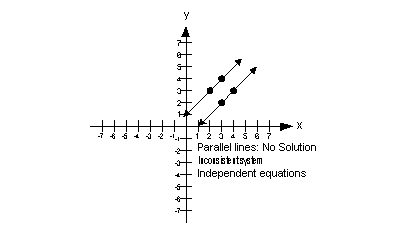Infinite Solutions
If the two lines end up lying on top of each other, then there is an infinite number of solutions.  In this situation, they would end up being the same line, so any solution that would work in one equation is going to work in the other.

If you get an infinite number of  solutions for your final answer, is this system consistent or inconsistent?
If you said consistent, you are right!

If you get an infinite number of  solutions for your final answer, would the equations be dependent or independent?
If you said dependent, you are correct!

The graph below illustrates a system of two equations and two unknowns that has an infinite number of solutions: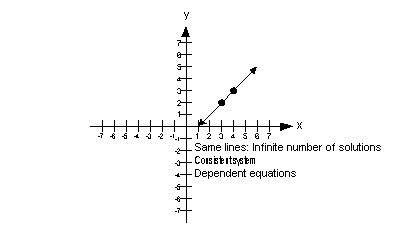Example 1:  Determine whether each ordered pair is a solution of the system.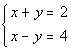(3, -1) and (0, 2)

Let’s check the ordered pair (3, -1) in the first equation: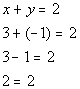*Plug in 3 for x and -1 for y

*True statement

So far so good,  (3, -1) is a solution to the first equation x + y = 2.

Now, let’s check (3, -1) in the second equation: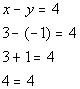*Plug in 3 for x and -1 for y

*True statement

Hey, we ended up with another true statement, which means (3, -1) is also a solution to the second equation x - y = 4.

Here is the big question, is (3, -1) a solution to the given system?????
Since it was a solution to BOTH equations in the system, then it is a solution to the overall system.

Now let’s put (0, 2) into the first equation: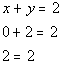*Plug in 0 for x and 2 for y
*True statement

This is a true statement, so (0, 2) is a solution to the first equation x + y = 2.

Finally, let’s put (0,2) into the second equation: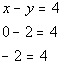*Plug in 0 for x and 2 for y
*False statement

This time we got a false statement, you know what that means.  (0, 2) is NOT a solution to the second equation x - y = 4.

Here is the big question, is (0, 2) a solution to the given system?????
Since it was not a solution to BOTH equations in the system, then it is not a solution to the overall system.

Three Ways to Solve Systems of Linear
Equations in Two Variables

There are three ways to solve systems of linear equations in two variables:

Solve by Graphing

Step 1: Graph the first equation.

Unless the directions tell you differently, you can use any "legitimate" way to graph the line.  Our tutorials show three different ways.  Feel free to review back over them if you need to:  Tutorial 12: Graphing Equations  shows how to graph by plotting points, Tutorial 14: Graphing Linear Equations shows how to graph using intercepts, and Tutorial 16: Equations of Lines shows how to graph using the y-intercept and slope.

Step 2: Graph the second equation on the same coordinate system as the first.

You graph the second equation the same as any other equation.  Refer to the first step if you need to review the different ways to graph a line.

The difference here is you will put it on the same coordinate system as the first.  It is like having two graphing problems in one.

Step 3: Find the solution.

If the two lines intersect at one place, then the point of intersection is the solution to the system.

If the two lines are parallel, then they never intersect, so there is no solution.

If the two lines lie on top of each other, then they are the same line and you have an infinite number of solutions..  In this case you can write down either equation as the solution to indicate they are the same line.

Step 4: Check the proposed ordered pair solution in BOTH equations.

You can plug in the proposed solution into BOTH equations.  If it makes BOTH equations true then you have your solution to the system.

If it makes at least one of them false, you need to go back and redo the problem.Example 2:  Solve the system of equations by graphing.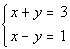Step 1: Graph the first equation.

x-intercept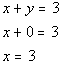*Plug in 0 for y for x-int
*x-intercept

The x-intercept is (3, 0).

y-intercept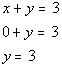*Plug in 0 for x for y-int
*y-intercept

The y-intercept is (0, 3).

Find another solution by letting x = 1.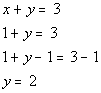*Plug in 1 for x
Another solution is (1, 2).

Solutions:

x y (x, y) 3 0 (3, 0) 0 3 (0, 3) 1 2 (1, 2)

Plotting the ordered pair solutions and drawing the line: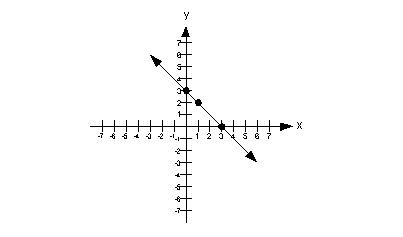Step 2: Graph the second equation on the same coordinate system as the first.

x-intercept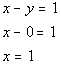*Plug in 0 for y for x-int
*x-intercept

The x-intercept is (1, 0).

y-intercept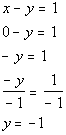*Plug in 0 for x for y-int

*Inverse of mult. by -1 is div. by -1

*y-intercept

The y-intercept is (0, -1).

Find another solution by letting x = 2.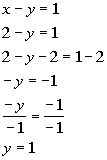*Plug in 2 for x
*Inverse of add 2 is sub. 2

*Inverse of mult. by -1 is div by -1

Another solution is (2, 1).

Solutions:

x y (x, y) 1 0 (1, 0) 0 -1 (0, -1) 2 1 (2, 1)

Plotting the ordered pair solutions and drawing the line: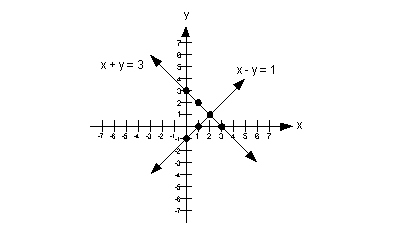Step 3: Find the solution.

We need to ask ourselves, is there any place that the two lines intersect, and if so, where?

The answer is yes, they intersect at (2, 1).

Step 4: Check the proposed ordered pair solution in BOTH equations.

You will find that if you plug the ordered pair (2, 1) into BOTH equations of the original system, that this is a solution to BOTH of them.

The solution to this system is (2, 1).Example 3:  Solve the system of equations by graphing.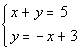Step 1: Graph the first equation.

x-intercept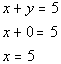*Plug in 0 for y for x-int
*x-intercept

The x-intercept is (5, 0).

y-intercept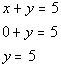*Plug in 0 for x for y-int

*y-intercept

The y-intercept is (0, 5).

Find another solution by letting x = 1.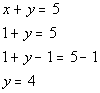*Plug in 1 for x
*Inverse of add 1 is sub. 1

Another solution is (1, 4).

Solutions:

x y (x, y) 5 0 (5, 0) 0 5 (0, 5) 1 4 (1, 4)

Plotting the ordered pair solutions and drawing the line: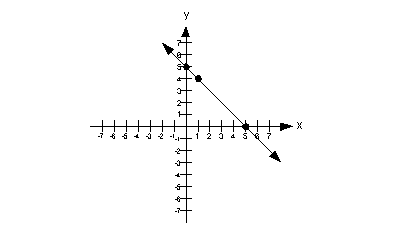Step 2: Graph the second equation on the same coordinate system as the first.

x-intercept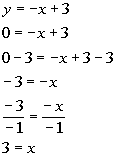*Plug in 0 for y for x-int
*Inverse of add 3 is sub. 3

*Inverse of mult. by -1 is div. by -1

*x-intercept

The x-intercept is (3, 0).

y-intercept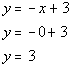*Plug in 0 for x for y-int
*y-intercept

The y-intercept is (0, 3).

Find another solution by letting x = 1.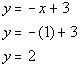*Plug in 1 for x

Another solution is (1, 2).

Solutions:

x y (x, y) 3 0 (3, 0) 0 3 (0, 3) 1 2 (1, 2)

Plotting the ordered pair solutions and drawing the line: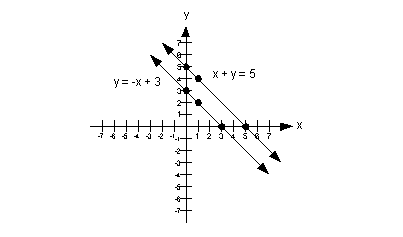Step 3: Find the solution.

We need to ask ourselves, is there any place that the two lines intersect, and if so, where?

The answer is no, they do not intersect.  We have two parallel lines.

Step 4: Check the proposed ordered pair solution in BOTH equations.

There are no ordered pairs to check.

Solve by the Substitution Method

Step 1: Simplify if needed.

This would involve things like removing ( ) and  removing fractions.

To remove ( ): just use the distributive property.

To remove fractions: since fractions are another way to write division, and the inverse of divide is to multiply, you remove fractions by multiplying both sides by the LCD of all of  your  fractions.

Step 2:  Solve one equation for either variable.

It doesn't matter which equation you use or which variable you choose to solve for.

You want to make it as simple as possible.  If one of the equations is already solved for one of the variables, that is a quick and easy way to go.

If you need to solve for a variable, then try to pick one that has a 1 as a coefficient.  That way when you go to solve for it, you won't have to divide by a number and run the risk of having to work with a fraction (yuck!!).

Step 3: Substitute what you get for step 2 into the other equation .

This is why it is called the substitution method.  Make sure that you substitute the expression into the OTHER equation, the one you didn't use in step 2.

This will give you one equation with one unknown.

Step 4:  Solve for the remaining variable .

Solve the equation set up in step 3 for the variable that is left.

If you need a review on this, go to Tutorial 7: Linear Equations in One Variable.

Step 5:  Solve for second variable.

If you come up with a value for the variable in step 4, that means the two equations have one solution.  Plug the value found in step 4 into any of the equations in the problem and solve for the other variable.

If your variable drops out and you have a FALSE statement, that means your answer is no solution.

If your variable drops out and you have a TRUE statement, that means your answer is infinite solutions, which would be the equation of the line.

Step 6: Check the proposed ordered pair solution in BOTH original equations.

You can plug in the proposed solution into BOTH equations.  If it makes BOTH equations true, then you have your solution to the system.

If it makes at least one of them false, you need to go back and redo the problem.Example 4:  Solve the system of equations by the substitution method.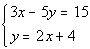Step 1: Simplify if needed.

Both of these equations are already simplified.  No work needs to be done here.

Step 2:  Solve one equation for either variable.

Note how the second equation is already solved for y.  We can use that one for this step.

It does not matter which equation or which variable you choose to solve for.  But it is to your advantage to keep it as simple as possible.

Second equation solved for y: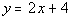*Solved for y

Substitute the expression 2x + 4 for y into  the first equation and solve for x:
(when you plug in an expression like this, it is just like you plug in a number for your variable)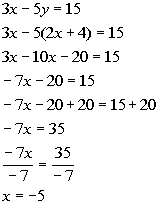*Sub. 2x + 4 in for y
*Dist. -5 through (  )
*Combine like terms

*Inverse of sub. 20 is add 20

*Inverse of div. by -7 is mult. by -7

Step 5:  Solve for second variable.

Plug in -5 for x into the equation in step 2 to find y’s value.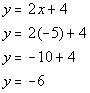*Plug in -5 for x

Step 6: Check the proposed ordered pair solution in BOTH original equations.

You will find that if you plug the ordered pair (-5, -6) into BOTH equations of the original system, that this is a solution to BOTH of them.

(-5, -6) is a solution to our system.Example 5:  Solve the system of equations by the substitution method.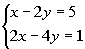Step 1: Simplify if needed.

Both of these equations are already simplified.  No work needs to be done here.

Step 2:  Solve one equation for either variable.

This time, the problem  was not so nice to us, we will have to do a little work to get one equation solved for one of our variables.

It does not matter which equation or which variable you choose to solve for.   Just keep it simple.

Since the x in the first equation has a coefficient of 1, that would mean we would not have to divide by a number to solve for it and run the risk of having to work with fractions (YUCK!!)  The easiest route here is to solve the first equation for x, and we definitely want to take the easy route.  You would not be wrong to either choose the other equation and/or solve for y, again you want to keep it as simple as possible.

Solving the first equation for x we get: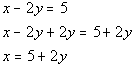*Inverse of sub. 2y is add 2y

*Solved for x

Substitute the expression 5 + 2 for x into the second equation and solve for y:
(when you plug in an expression like this, it is just like you plug in a number for your variable)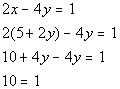*Sub. 5 + 2y for x

*Variable dropped out AND false

Wait a minute, where did our variable go????

As mentioned above if your variable drops out and you have a FALSE statement, then there is no solution.  If we were to graph these two, they would be parallel to each other.

Step 5:  Solve for second variable.

Since we did not get a value for y, there is nothing to plug in here.

Step 6: Check the proposed ordered pair solution in BOTH original equations.

There are no ordered pairs to check.

Solve by the Elimination Method

This methods is also known as the addition or the elimination by addition method.

Step 1: Simplify and put both equations in the form Ax + By = C if needed.

This would involve things like removing ( ) and  removing fractions.

To remove ( ): just use the distributive property.

To remove fractions: since fractions are another way to write division, and the inverse of divide is to multiply, you remove fractions by multiplying both sides by the LCD of all of  your  fractions.

Step 2: Multiply one or both equations by a number that will create opposite coefficients for either x or y if needed.

Looking ahead, we will be adding these two equations together.  In that process, we need to make sure that one of the variables drops out, leaving us with one equation and one unknown.  The only way we can guarantee that is if we are adding opposites.  The sum of opposites is 0.

If neither variable drops out, then we are stuck with an equation with two unknowns which is unsolvable.

It doesn't matter which variable you choose to drop out.  You want to keep it as simple as possible.  If a variable already has opposite coefficients than go right to adding the two equations together.  If they don't, you need to multiply one or both equations by a number that will create opposite coefficients in one of your variables.  You can think of it like a LCD.  Think about what number the original coefficients both go into and multiply each separate equation accordingly.  Make sure that one variable is positive and the other is negative before you add.

For example, if you had a 2x in one equation and a 3x in another equation, we could multiply the first equation by 3 and get 6x and the second equation by -2 to get a -6x.  So when you go to add these two together they will drop out.

The variable that has the opposite coefficients will drop out in this step and you will be left with one equation with one unknown.

Step 4: Solve for remaining variable.

Solve the equation found in step 3 for the variable that is left.

If you need a review on this, go to Tutorial 7: Linear Equations in One Variable.

If both variables drop out and you have a FALSE statement, that means your answer is no solution.

If both variables drop out and you have a TRUE statement, that means your answer is infinite solutions, which would be the equation of the line.

Step 5: Solve for second variable.

If you come up with a value for the variable in step 4, that means the two equations have one solution.  Plug the value found in step 4 into any of the equations in the problem and solve for the other variable.

Step 6: Check the proposed ordered pair solution in BOTH original equations.

You can plug the proposed solution into BOTH equations.  If it makes BOTH equations true, then you have your solution to the system.

If it makes at least one of them false, you need to go back and redo the problem.Example 6:  Solve the system of equations by the elimination method.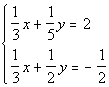Step 1: Simplify and put both equations in the form Ax + By = C if needed.

This equation is full of those nasty fractions.  We can simplify both equations by multiplying each separate one by it’s LCD, just like you can do when you are working with one equation.  As long as you do the same thing to both sides of an equation, you keep the two sides equal to each other.

Multiplying each equation by it's respective LCD we get: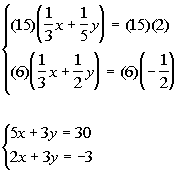*Mult. by LCD of 15

*Mult. by LCD of 6

Step 2: Multiply one or both equations by a number that will create opposite coefficients for either x or y if needed.

Again, you want to make this as simple as possible.  Note how the coefficients on both y’s are 3.  We need to have opposites, so if one of them is 3 and the other is -3, they would cancel each other out when we go to add them.

If we added them together the way they are now, we would end up with one equation and two variables, nothing would drop out.  And we would not be able to solve it.

I propose that we multiply the second equation by -1, this would create a -3 in front of x and we will have our opposites.

Note that we could just as easily multiply the first equation by -1 and not the second one.  Either way will get the job done.

Multiplying the second equation by -1 we get: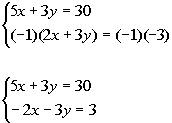*Mult. both sides of 2nd eq. by -1

*y's have opposite coefficients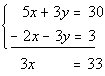*Note that y's dropped out

Step 4: Solve for remaining variable.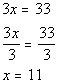*Inverse of mult. by 3 is div. by 3

Step 5: Solve for second variable.

You can choose any equation used in this problem to plug in the found x value.

I choose to plug in 11 for x into the first simplified equation (found in step 1) to find y’s value.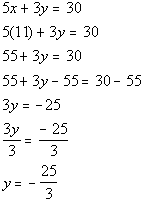*Plug in 11 for x

*Inverse of add 55 is sub. 55

*Inverse of mult. by 3 is div. by 3

Step 6: Check the proposed ordered pair solution in BOTH original equations.

You will find that if you plug the ordered pair (11, -25/3) into BOTH equations of the original system, that this is a solution to BOTH of them.

(11, -25/3) is a solution to our system.Example 7:  Solve the system of equations by the elimination method.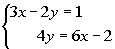Step 1: Simplify and put both equations in the form Ax + By = C if needed.

This problem is already simplified. However, the second equation is not written in the form Ax + By = C.  In other words, we need to write it in this form so everything is lined up ready to go when we add the two equations together.

Rewriting the second equation we get: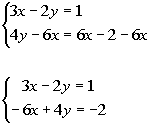*Inverse of add 6x is sub. 6x

*Everything lines up

Step 2: Multiply one or both equations by a number that will create opposite coefficients for either x or y if needed.

Note that if we multiply the first equation by 2, then we will have a -6x which is the opposite of the 6x found in the second equation.

Multiplying the first equation by 2 we get: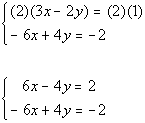*Mult. 1st eq. by 2

*x's have opposite coefficients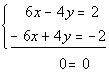*Variables dropped out AND true

Step 4: Solve for remaining variable.

Hey where did our variables go??

As mentioned above, if the variable drops out AND we have a TRUE statement, then when have an infinite number of solutions.  They end up being the same line.

Step 5: Solve for second variable.

There is no value to plug in here.

Step 6: Check the proposed ordered pair solution in BOTH original equations.

There is no value to plug in here.

When they end up being the same equation, you have an infinite number of solutions.  You can write up your answer by writing out either equation to indicate that they are the same equation.

Two ways to write the answer are {(x, y)| 3x - 2y = 1} OR {(x, y) | 4y = 6x - 2}.Practice Problems

These are practice problems to help bring you to the next level.  It will allow you to check and see if you have an understanding of these types of problems. Math works just like anything else, if you want to get good at it, then you need to practice it.  Even the best athletes and musicians had help along the way and lots of practice, practice, practice, to get good at their sport or instrument.  In fact there is no such thing as too much practice.

To get the most out of these, you should work the problem out on your own and then check your answer by clicking on the link for the answer/discussion for that  problem.  At the link you will find the answer as well as any steps that went into finding that answer.Practice Problem 1a: Solve the system by graphing.

1a.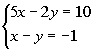Practice Problem 2a: Solve the system by the substitution method.

2a.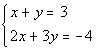Practice Problem 3a: Solve the system by the elimination method.

3a.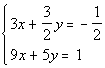Need Extra Help on these Topics?

The following is a website that can assist you in the topics that were covered on this page:

http://www.purplemath.com/modules/systlin1.htm
This website helps you with the definition of and solving systems of linear equations.

Go to Get Help Outside the Classroom found in Tutorial 1: How to Succeed in a Math Class for some more suggestions.Last revised on July 10, 2011 by Kim Seward.